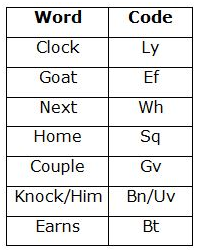# IBPS Clerk Prelims Reasoning Ability Questions 2021 (Day-01)

Dear Aspirants, Our IBPS Guide team is providing new series of Reasoning Questions for IBPS Clerk Prelims 2021 so the aspirants can practice it on a daily basis. These questions are framed by our skilled experts after understanding your needs thoroughly. Aspirants can practice these new series questions daily to familiarize with the exact exam pattern and make your preparation effective.

Start Quiz

Alphanumeric series

Direction (1-5): Study the following information to answer the given questions:

P 4 % L K U N * 7 B G 9 \$ V F O @ 2 D I E 3 ¬ W S & Z A £ 5 C Z 1 X

1) How many such vowels are there which is immediately preceded by a consonant and immediately followed by a symbol?

A.One

B.Two

C.Three

D.Four

E.None of these

2) Which of the following element is exactly between 14th  element from the left end and 11th element from the right end?

A.I

B.E

C.2

D.D

E.None of these

3) How many such numbers are there which is immediately preceded by a symbol and immediately followed by a consonant?

A.One

B.Two

C.Three

D.Four

E.None of these

4) Which of the following element is thirteenth to the left of eighth from the right end?

A.\$

B.V

C.F

D.9

E.None of these

5) If all the numbers are removed from the given series, then which of the following vowel is fifth from the left end?

A.E

B.U

C.A

D.I

E.None

Coding decoding

Direction (6-10): Study the following information carefully and answer the questions given below:

In a certain code language:

“Next goat clock earns” is coded as “wh bt ly ef”

“Earns home knock him” is coded as “uv bn bt sq”

“Goat knock him next” is coded as “wh bn uv ef”

“Clock goat couple home” is coded as “sq ef ly gv”

6) Which of the following is the code for “Home earns”?

A.bt sq

B.bt uv

C.sq bn

D.bt ly

E.None of these

7) Which of the following is the code for “Knock”?

A.uv

B.bt

C.sq

D.bn

E.Either a) or d)

8) If “see him” is coded as “fr bn” then which of the following can be the code of “Knock bring”?

A.uv sq

B.bn wh

C.ef uv

D.uv rg

E.bt uv

9) Which of the following is the code for “Couple him earns”?

A.bt gv uv

B.gv bt bn

C.bn uv bt

D.gv bn uv

E.Can’t be determined

10) Which of the following is the code for “Clock”?

A.ef

B.wh

C.sq

D.ly

E.bt

[email protected], ZA£

P 4 % L K U N * 7 B G 9 \$ V F O @ 2 D I E 3 ¬ W S & Z A £ 5 C Z 1 X

*7B,@2D, £5C

13+8=21st from the right end is V

P % L K U N *  B G  \$ V F O @ D I E ¬ W S & Z A £ C Z X

Directions (6-10) :